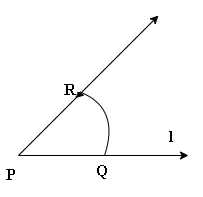Courses
Courses for Kids
Free study material
Offline Centres
More

# Practical Geometry Class 6 Notes CBSE Maths Chapter 14 (Free PDF Download)Last updated date: 27th Nov 2023
Total views: 589.8k
Views today: 11.89k

## Revision Notes for CBSE Class 6 Maths Chapter 14 - Free PDF Download

Free PDF download of Class 6 Maths Chapter 14 - Practical Geometry Revision Notes & Short Key-notes prepared by expert Maths teachers from latest edition of CBSE(NCERT) books. To register Maths Tuitions on Vedantu.com to clear your doubts.

## Access Class 6 Mathematics Chapter 14 - Practical Geometry

The methods for sketching geometrical forms are covered in this chapter.

• To create shapes, we employ the following mathematical instruments:

• Along one edge, a ruler graduated in centimetres (and sometimes into inches along the other edge).

• It is used to draw and measure the line segments.

1. The compass:

• It is a pair of a pencil on one end and a pointer on the other.

• It is used not to measure the equal lengths, but to mark them off.

• Also, it is used to make circles and arcs.

1. The divider:

• It is a pair of pointers.

• It is used to compare the lengths.

1. Set-squares:

• Set squares are the two triangular pieces, one with ${{45}^{0}},{{45}^{0}}$ and ${{90}^{0}}$ angles at the vertices and the other with ${{30}^{0}},{{60}^{0}}$ and ${{90}^{0}}$ angles.

• It is used to draw the parallel and perpendicular lines.

1. The protractor:

• It is used to measure the angles.

• It is like a semi-circular scale with markings as angles.

• The following constructions can be created with the ruler and compass:

1. A circle can be drawn only when the length of its radius is known.

2. A line segment can be drawn when its length is given.

3. Same procedure follows for the line segment.

4. A perpendicular to a line can be drawn through a point

1. On the line

2. Not on the line.

1. The perpendicular bisector of a line segment of given length can be drawn.

2. An angle can be drawn for a given measure.

3. A copy of an angle.

4. The bisector of a given angle.

5. Some angles of special measures such as

1. ${{90}^{0}}$

2. ${{45}^{0}}$

3. ${{60}^{0}}$

4. ${{30}^{0}}$

5. ${{120}^{0}}$

6. ${{135}^{0}}$

Construction of a circle when its radius is known:

Step $1$:

Open the compass for the required radius.

Step $2$:

Mark a point with a sharp pencil to denote where the centre of the circle has to be. Name it as $O$.

Step $3$:

Place the pointer of the compass on $O$.

Step $4$:

Now, turn the compass slowly either in clockwise or anticlockwise direction such that the pencil traces the circle of required radius. Care must be taken to complete the movement at one go.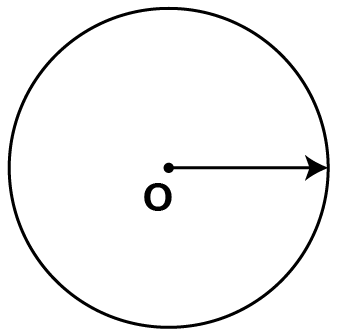Construction of a line segment of a given length:

A better method would be to construct a line segment of a given length with a compass.

Draw a line for $\text{3 cm}$ by using the following steps.

Step $1$:

Draw a line $l$ and make a point $A$ on line $l$.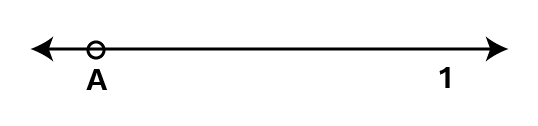Step $2$:

Place the pointer of the compass at the zero mark of the ruler. Extend the other leg of the compass upto the 3 cm mark on the ruler.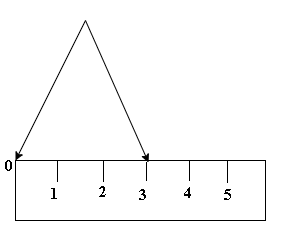Step $3$:

Taking caution that the opening of the compass has not changed, place the pointer on $A$ and swing an arc to cut $l$ at $B$.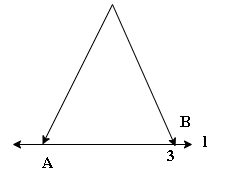Step $4$:

$\overline{AB}$ is a line segment of required length.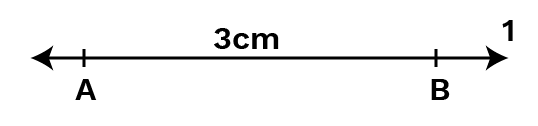### Constructing a Copy of a Given Line Segment:

A better technique would be to construct a line segment with a ruler and compass.

Following steps show how to draw $\overline{AB}$.

Step $1$:

Given $\overline{AB}$ whose length is not known.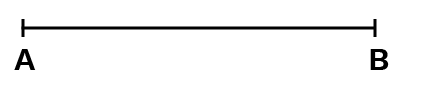Step $2$:

Fix the compass pointer on $A$ and the pencil end on $B$.

The distance between the two open legs of the instrument now gives the length of $\overline{AB}$.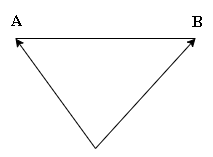Step $3$:

Draw any line $l$.

Choose a point $C$ on $l$.

Without changing the compass setting, place the pointer on $C$.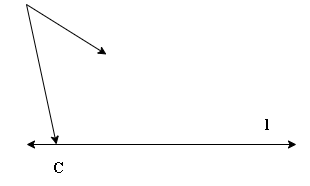Step $4$:

Swing an arc that cuts $l$ at a point, say, $D$.

Now $\overline{CD}$ is a copy of $\overline{AB}$.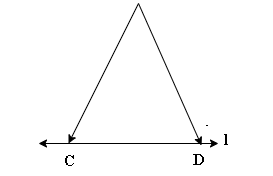### Method of Ruler and Compass:

Step $1$:

Given a point $P$ on a line $l$.Step $2$:

With $P$ as centre and a convenient radius, construct an arc intersecting the line $l$ at two points $A$ and $B$.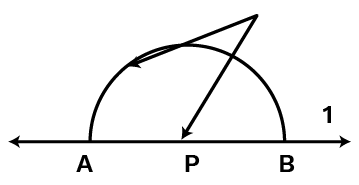Step $3$:

With $A$ and $B$ as centres and a radius greater than $AP$ construct two arcs, which cut each other at $Q$.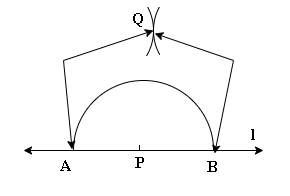Step $4$:

Join $PQ$.

Then $\overline{PQ}$ is perpendicular to $l$.

We write $\overline{PQ}\bot l$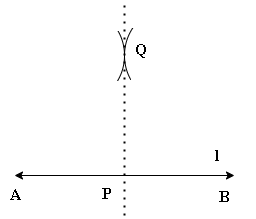### Constructing a Copy of an Angle of Unknown Measure:

We have to use only a straightedge and the compass for constructing an angle whose measure is unknown.

With the help of following steps, we can draw an unknown angle $\angle A$

Step $1$:

Draw a line $l$ and choose a point $P$ on it.Step $2$:

Place the compass at $A$ and draw an arc to cut the rays of $\angle A$ at $B$ and $C$.Step $3$:

Maintaining the same settings on the compass, draw an arc with $P$ as centre, cutting $l$ in $Q$.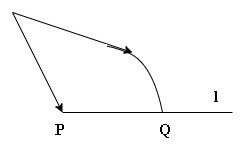Step $4$:

Set the compass to the length $BC$ with the same radius.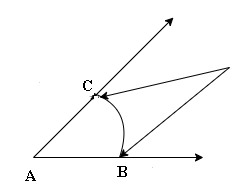Step $5$:

Place the compass pointer at $Q$ and draw the arc to cut the arc drawn earlier in $R$.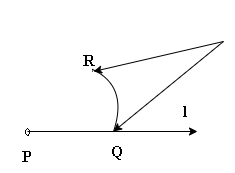Step $6$:

Join $PR$. This gives us $\angle P$ . It has the same measure as $\angle A$ .

This means $\angle QPR$ has the same measure as $\angle BAC$.